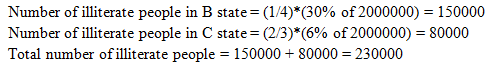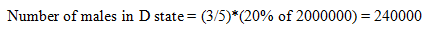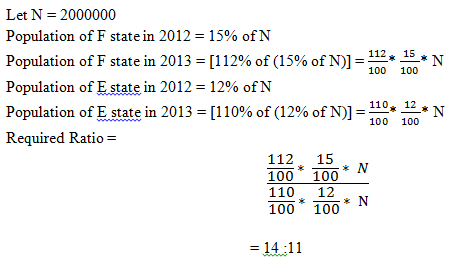# High Level Data Interpretation Questions For IBPS PO 2017: Part II

Question (1-5) : Study the following graph and the table and answer the questions given below:
Data of different states regarding population of states in the year 2012

Total population of the given states = 2000000.

States
Sex and Literacy wise Population Ratio
Sex
Literacy
Male : Female
Literate : Illiterate
A
1 : 4
2 : 5
B
3 : 2
3 : 1
C
3 : 5
1 : 2
D
3 : 2
5 : 1
E
2 : 3
2 : 1
F
1 : 3
1 : 4
G
2 : 1
2 : 3

1) What was the total number of illiterate people in B state and C state in 2012?
A. 225000
B. 150000
C. 230000
D. 330000
E. None of these

2) What was the number of males in D state in the year 2012?
A. 220000
B. 120000
C. 260000
D. 240000
E. None of these

3) In the year 2013, there was an increase of 12% in the population of F state and 10% in the population of E state compared to 2012, what was the ratio of F state and E state in 2013?
A. 14:11
B. 11:10
C. 13:14
D. 15:13
E. Can not be determined

4) What is the ratio of the number of females in G states to the number of females in B state?
A. 2:3
B. 1:3
C. 1:4
D. 2:5
E. 3:2

5) What will be the percentage of the total number of males in states A,C and E together to the total population of all the given states?
A. 9.05%
B. 10.3%
C. 6.67%
D. 7.49%
E. 8.85%

#### Ans 1.#### Ans 2.#### Ans 3.#### Ans 4.#### Ans 5.#### What's trending in BankExamsToday

Smart Prep Kit for Banking Exams by Ramandeep Singh - Download here